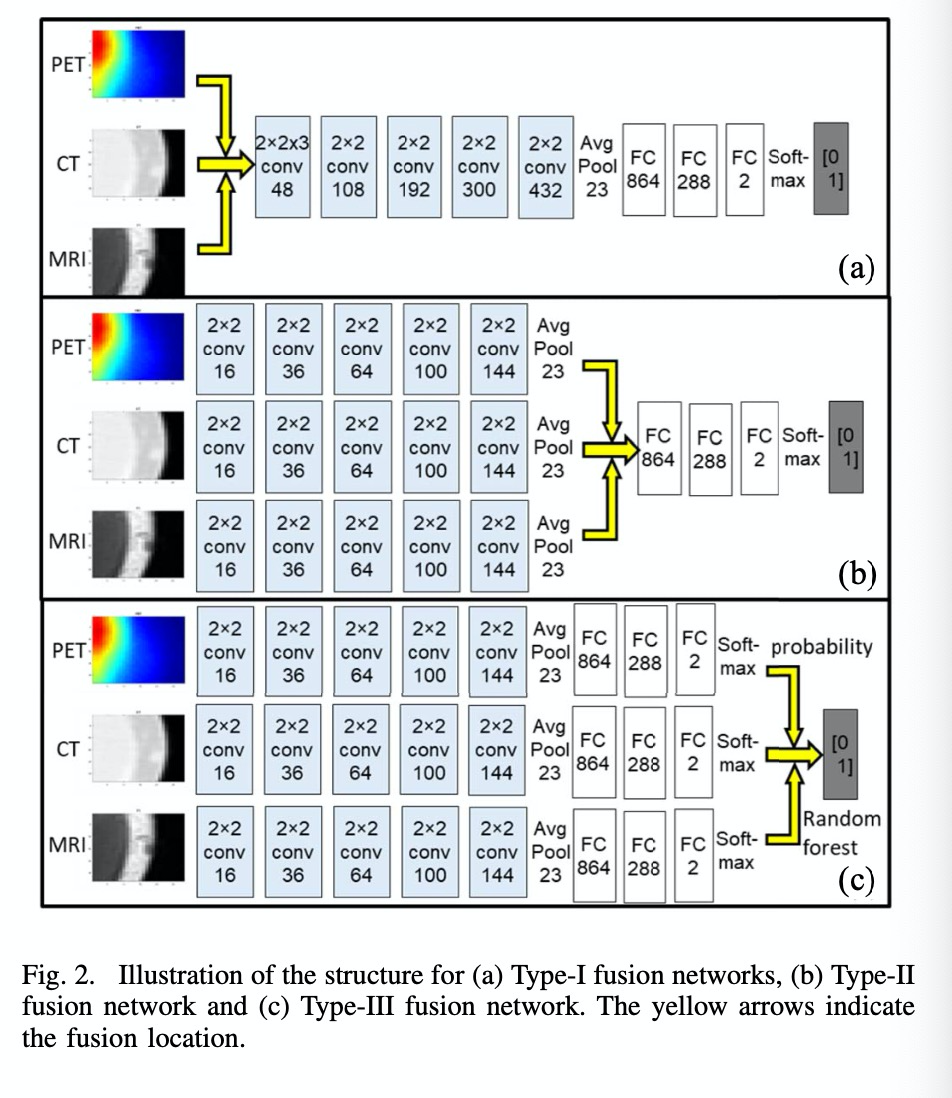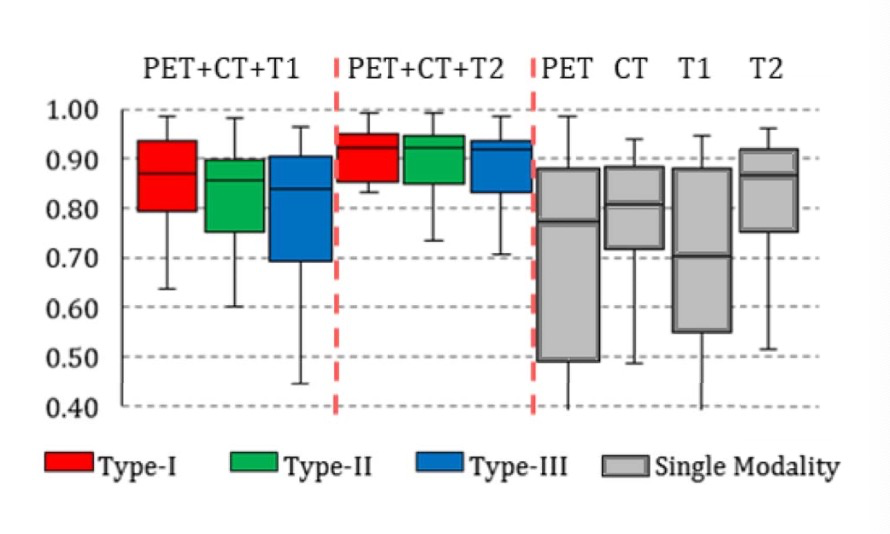# 1.引言# 2.简要解析np.concatente官方文档

`numpy.``concatenate`((a1a2…)axis=0out=None)

Join a sequence of arrays along an existing axis.

Parameters: a1, a2, … : sequence of array_like The arrays must have the same shape, except in the dimension corresponding to axis (the first, by default). axis : int, optional The axis along which the arrays will be joined. If axis is None, arrays are flattened before use. Default is 0. out : ndarray, optional If provided, the destination to place the result. The shape must be correct, matching that of what concatenate would have returned if no out argument were specified. res : ndarray The concatenated array.

concatenate中含有两个参数，第一个参数是一个元组，元组里填入你想合并的数组，第二个参数是axis轴，第一个参数没什么好说的，主要想谈第二个参数。

`>>> a = np.array([[1, 2], [3, 4]])  >>> b = np.array([[5, 6]])  >>> np.concatenate((a, b), axis=0)  array([[1, 2],         [3, 4],         [5, 6]])  >>> np.concatenate((a, b.T), axis=1)  array([[1, 2, 5],         [3, 4, 6]])  >>> np.concatenate((a, b), axis=None)  array([1, 2, 3, 4, 5, 6])  `

`>>> a = np.ma.arange(3)  >>> a = np.ma.masked  >>> b = np.arange(2, 5)  >>> a  masked_array(data = [0 -- 2],               mask = [False  True False],         fill_value = 999999)  >>> b  array([2, 3, 4])  >>> np.concatenate([a, b])  masked_array(data = [0 1 2 2 3 4],               mask = False,         fill_value = 999999)  >>> np.ma.concatenate([a, b])  masked_array(data = [0 -- 2 2 3 4],               mask = [False  True False False False False],         fill_value = 999999)  `

# 3.axis的轴到底指的是什么

`a  array(［0, 1, 2],         [3, 4, 5],         [6, 7, 8］)  b = a*2  b  array(［ 0, 2, 4],         [ 6, 8, 10],         [12, 14, 16］)  `

下面进行合并：

`np.hstack((a,b))  array(［ 0, 1, 2, 0, 2, 4],         [ 3, 4, 5, 6, 8, 10],         [ 6, 7, 8, 12, 14, 16］)    np.concatenate((a,b),axis=1)  array(［ 0, 1, 2, 0, 2, 4],         [ 3, 4, 5, 6, 8, 10],         [ 6, 7, 8, 12, 14, 16］)`
`np.vstack((a,b))  array(［ 0, 1, 2],         [ 3, 4, 5],         [ 6, 7, 8],         [ 0, 2, 4],         [ 6, 8, 10],         [12, 14, 16］)    np.concatenate((a,b),axis=0)  array(［ 0, 1, 2],         [ 3, 4, 5],         [ 6, 7, 8],         [ 0, 2, 4],         [ 6, 8, 10],         [12, 14, 16］)`

两种方式分别和np中的hstack以及vstack的合并数组的结果相同，那么concatenate存在的意义到底是什么呢，就在于它可以进行更高维度的数组合并。

`#coding=utf-8  import numpy as np    np.random.seed(0)  a = np.random.randint(0, 255, (10, 1, 128, 128))  b = np.random.randint(0, 255, (10, 1, 128,128))  ab = np.concatenate((a,b),axis = 1)  print('ab的维度为 = n {}'.format(ab.shape))ab的维度为 =   (10, 2, 128, 128)  `